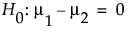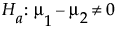Publication date: 04/12/2021

# Two Sample Means Calculator

Use the Two Sample Means calculator to evaluate sample size for a hypothesis test about two means. Explore questions such as how large a treatment effect is detectable for a given sample size, significance level, desired power, and assumed variability in the data. Sample size and power are associated with the following hypothesis test:versus the two-sided alternative:where μ1 and μ2 are the true means of the two populations. It is assumed that the populations of interest are normally distributed and that you want to detect a difference of δ between the means.

Want more information? Have questions? Get answers in the JMP User Community (community.jmp.com).